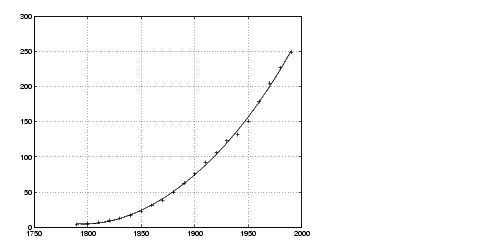MathematicsPolynomial Fit

A first try in fitting the census data might be a simple polynomial fit. Two MATLAB functions help with this process.

 Function Description `polyfit` Polynomial curve fit. `polyval` Evaluation of polynomial fit.

The MATLAB `polyfit` function generates a "best fit" polynomial (in the least squares sense) of a specified order for a given set of data. For a polynomial fit of the fourth-order

• ```p = polyfit(cdate,pop,4)
Warning: Polynomial is badly conditioned. Remove repeated data
points or try centering and scaling as described in HELP POLYFIT.

p =
1.0e+05 *

0.0000   -0.0000    0.0000   -0.0126 6.0020
```

The warning arises because the `polyfit` function uses the `cdate` values as the basis for a matrix with very large values (it creates a Vandermonde matrix in its calculations - see the `polyfit` M-file for details). The spread of the `cdate` values results in scaling problems. One way to deal with this is to normalize the `cdate` data.

Preprocessing: Normalizing the Data

Normalization is a process of scaling the numbers in a data set to improve the accuracy of the subsequent numeric computations. A way to normalize `cdate` is to center it at zero mean and scale it to unit standard deviation:

• ```sdate = (cdate - mean(cdate))./std(cdate)
```

Now try the fourth-degree polynomial model using the normalized data:

• ```p = polyfit(sdate,pop,4)

p =
0.7047    0.9210   23.4706   73.8598   62.2285
```

Evaluate the fitted polynomial at the normalized year values, and plot the fit against the observed data points:

• ```pop4 = polyval(p,sdate);
plot(cdate,pop4,'-',cdate,pop,'+'), grid on```

Another way to normalize data is to use some knowledge of the solution and units. For example, with this data set, choosing 1790 to be year zero would also have produced satisfactory results.Case Study: Curve Fitting Analyzing Residuals© 1994-2005 The MathWorks, Inc.